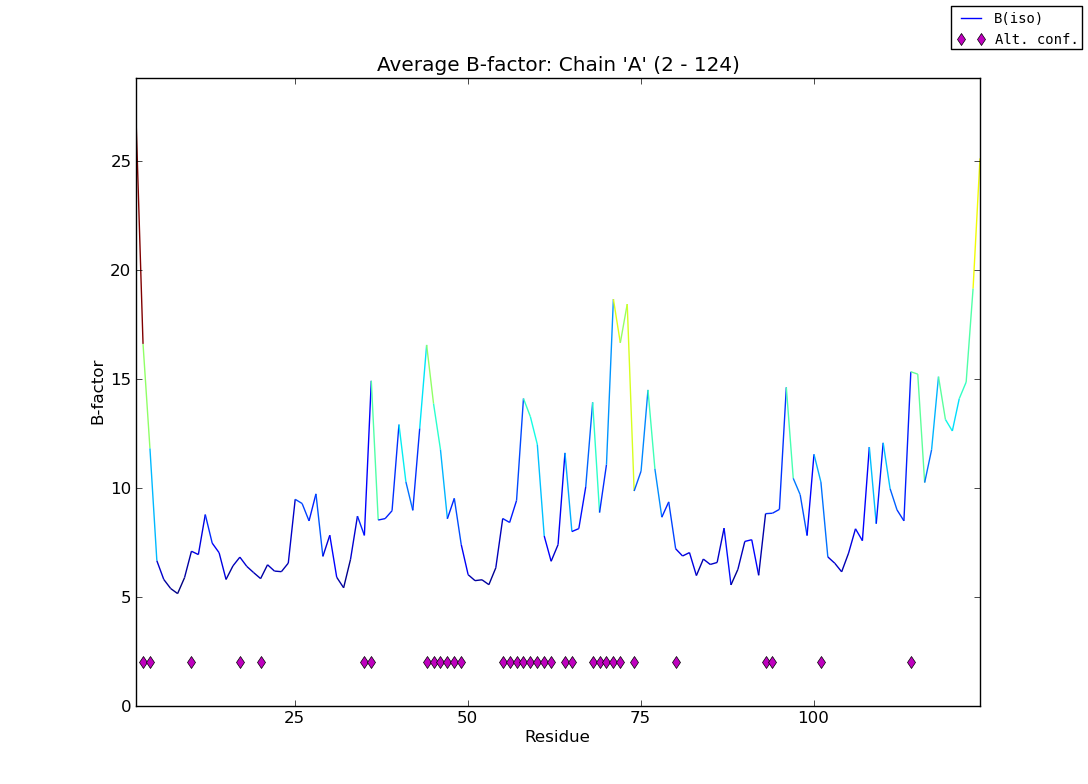# applying colormap to a line

This works brilliantly, although I’m still not sure what some of these API calls do. Now a simpler problem: the color of each line depends on the starting Y value (see attached image). The effect is especially noticeable when there is a large change in value. I’d like to divide the line segments up to get a smoother color gradient, but the values are dictated by the experiment, not a mathematical function. My code is basically as follows:

y = numpy.array(avg_b)

x = numpy.linspace(1, y.size, y.size)

points = numpy.array([x, y]).T.reshape(-1,1,2)

yy = numpy.linspace(y.min(), y.max(), cm.jet.N)

norm = BoundaryNorm(yy, 256)

segments = numpy.concatenate([points[:-1], points[1:]], axis=1)

lc = LineCollection(segments, cmap=cm.jet, norm=norm)

lc.set_array(y)

so I guess what I’m really asking for is a way to add intermediate X,Y values between every pair of values in ‘points’. I can do this myself in Python, but I assume that’s going to be pretty sluggish.

thanks,

Nat···

On Thu, Mar 31, 2011 at 5:30 AM, <matplotlib-users-request@…642…ts.sourceforge.net> wrote:

On 03/30/2011 01:32 PM, Nat Echols wrote:

I wanted to display a line plot with rainbow coloring based on the

y-value, similar to what’s possible for surface plots. However, the

‘plot’ method does not appear to accept a ‘cmap’ argument. The closest

thing I was able to find was a recipe for different colored line

segments on the SciPy examples page

not really what I want - I was hoping for a continuous gradient over

hundreds (possibly thousands) of points on a line. Is this possible

without too much hacking?

I don’t think there is anything better than the second example here:

http://matplotlib.sourceforge.net/examples/pylab_examples/multicolored_line.html

From: Nat Echols [mailto:nathaniel.echols@…287…]
Sent: Thursday, March 31, 2011 16:47

I’d like to divide the line segments up to get a smoother color gradient, but the values are dictated by the experiment, not a mathematical function.

so I guess what I’m really asking for is a way to add intermediate X,Y values between every pair of values in ‘points’. I can do this myself in Python, but I assume that’s going to be pretty sluggish.

Perhaps (for convenience if not speed) you could use a routine from scipy.interpolate [1,2], such as interp1d for piecewise linear interpolation. I imagine that, within each linear piece, you would want the density of x values to be roughly proportional to the slope.